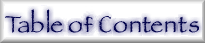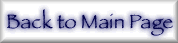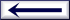## 16. Explanation of Tables and Tabulated Figures

Form factors, attenuation and scattering cross-sections, Z = 1-92, from E = 0.001-0.010 keV to E = 400-1000 keV.
 Z Atomic number E Photon energy in keV λ Equivalent wavelength using E ·λ = 1.23984244 keV-nm, from Ref.  Atomic weight g/mol, used in determination of conversion factors ρ Nominal density in typical elemental material for users g/cm3 µa Conversion for photoabsorption to barns/atom Edge energies Values taken from Ref.  Edge labels Spectroscopic notation (K=1s, LI=2s, LII=2p1/2, et seq.) f1, f2 Atomic scattering factors in electrons/atom (or eu) for forward scattering using (eq 3-7) and (eq 8-18) frel Relativistic correction to Re(f), following Ref.  (denoted H82), and Ref. [17-20] scaled as in Ref. [38,39] (denoted 3/5CL) fNT Nuclear Thomson correction to Re(f) following (eq 4) and Ref. [13,14] f2, K-shell Component of f2 relating to the isolated K-shell orbital µ = µPE Photoabsorption cross-section in cm2/g µK Component of µ relating to the isolated K-shell orbital σ(coh+inc) = σcoh+σinc Estimate of coherent and incoherent scattering cross-section sum Attenuation coefficient  = µ + σ(coh+inc) Attenuation coefficient in cm2/gm Full lines on plots Current tabulation (extended) for f1, f2, and µ Dot-dash curves Results reinterpolated from Ref. [15-16], for f1, f2, and µ Dash in f2 plots f2 K-shell Dash in µ plots σ(coh+inc)